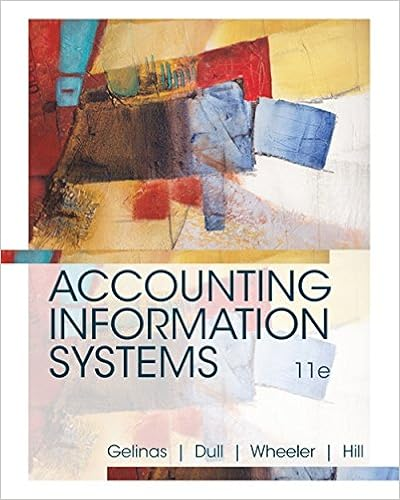Diff 2 lo 6 2 aacsb application of knowledge aicpa

• Assessment
• 152
• 92% (1194) 1098 out of 1194 people found this document helpful

This preview shows page 123 - 126 out of 152 pages.

We have textbook solutions for you!
The document you are viewing contains questions related to this textbook.The document you are viewing contains questions related to this textbook.
Chapter 15 / Exercise P15-6
Accounting Information Systems
Dull/GelinasExpert Verified
Diff: 2 LO: 6-2 AACSB: Application of knowledge AICPA Functional: Measurement
We have textbook solutions for you!
The document you are viewing contains questions related to this textbook.The document you are viewing contains questions related to this textbook.
Chapter 15 / Exercise P15-6
Accounting Information Systems
Dull/GelinasExpert Verified
PE Question Type: Application H2: Last-In, First-Out (LIFO) Method 46) Centennial, Inc. sold 600 units of inventory at \$20 per unit on account. The company uses the perpetual inventory system and the last-in, first-out (LIFO) inventory costing system. The beginning inventory included 200 units at \$9 per unit. The most recent purchases include 700 units at \$12 per unit. The sale occurred after the last purchase. a. Prepare the journal entries to record the sale. Omit explanations. b. Compute the cost of the ending inventory. Label your work. Answer: a. Cash 12,000 Sales Revenue 12,000 Cost of Goods Sold 7,200 Merchandise Inventory 7,200 Cost of goods sold = 600 units × \$12 per unit b. Ending inventory = beginning inventory (200 units × \$9 per unit) + most recent purchases (100 units × \$12 per unit) Ending inventory = \$1,800 + \$1,200 Ending inventory = \$3,000 47) Designer Furniture began June with merchandise inventory of 45 sofas that cost a total of \$31,500. During the month, Designer Furniture purchased and sold merchandise on account as follows: June 7 Purchase 25 sofas @ \$750 each 14 Sale 30 sofas @ \$1,150 each 18 Purchase 50 sofas @ \$775 each 27 Sale 35 sofas @ \$1,200 each Prepare a perpetual inventory record, using the LIFO inventory costing method, and determine the company's cost of goods sold, ending merchandise inventory, and gross profit. | Purchases | Cost of Goods Sold | Inventory on Hand | Date Quant Unit Cost Total Cost Quant Unit Cost Total Cost Quant Unit Cost Total Cost Answer:
Gross Profit = Sales - Cost of Goods Sold Gross Profit = \$76,500 - \$49,375 = \$27,125 Sales = (30 × \$1,150) + (35 × \$1,200) = \$76,500 48) When using the weighted-average inventory costing method in a perpetual inventory system, a new weighted average cost per unit is computed at the end of each quarter. Answer: FALSE 49) Weighted average cost per unit is determined by dividing the cost of goods available for sale by the number of units available. Answer: TRUE 50) The Organizer Store uses the weighted-average inventory costing method in a perpetual inventory system. The unit cost of the beginning inventory for inventory item X500 was \$10 per unit and the first purchase of the period has a unit cost of \$12 per unit. The weighted average cost per unit is \$11. Assume multiple units were purchased. Answer: FALSE Explanation: To compute the weighted average cost per unit it is necessary to use the total cost of goods available for sale divided by the number of units available for sale. Diff: 1 LO: 6-2 AACSB: Application of knowledge AICPA Functional: Measurement PE Question Type: Application H2: Weighted-Average Method 51) Which of the following inventory costing methods requires the calculation of a new average cost after each purchase? A) specific identification B) weighted-average C) last-in, first-out D) first-in, first-out Answer: B
•••